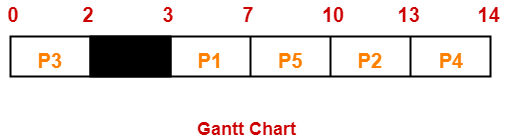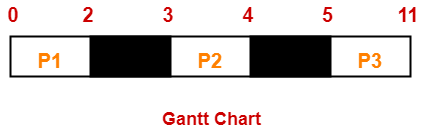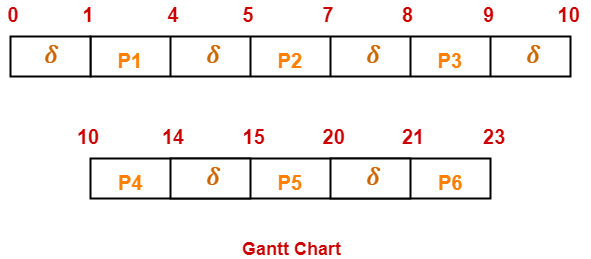# First Come First Serve | CPU Scheduling

## FCFS Scheduling-

In FCFS Scheduling,

• The process which arrives first in the ready queue is firstly assigned the CPU.
• In case of a tie, process with smaller process id is executed first.
• It is always non-preemptive in nature.

• It is simple and easy to understand.
• It can be easily implemented using queue data structure.
• It does not lead to starvation.

• It does not consider the priority or burst time of the processes.
• It suffers from convoy effect.

### Convoy Effect

In convoy effect,

• Consider processes with higher burst time arrived before the processes with smaller burst time.
• Then, smaller processes have to wait for a long time for longer processes to release the CPU.

## Problem-01:

Consider the set of 5 processes whose arrival time and burst time are given below-

 Process Id Arrival time Burst time P1 3 4 P2 5 3 P3 0 2 P4 5 1 P5 4 3

If the CPU scheduling policy is FCFS, calculate the average waiting time and average turn around time.

## Solution-

### Gantt Chart-Here, black box represents the idle time of CPU.

Now, we know-

• Turn Around time = Exit time – Arrival time
• Waiting time = Turn Around time – Burst time

Also read- Various Times of Process

 Process Id Exit time Turn Around time Waiting time P1 7 7 – 3 = 4 4 – 4 = 0 P2 13 13 – 5 = 8 8 – 3 = 5 P3 2 2 – 0 = 2 2 – 2 = 0 P4 14 14 – 5 = 9 9 – 1 = 8 P5 10 10 – 4 = 6 6 – 3 = 3

Now,

• Average Turn Around time = (4 + 8 + 2 + 9 + 6) / 5 = 29 / 5 = 5.8 unit
• Average waiting time = (0 + 5 + 0 + 8 + 3) / 5 = 16 / 5 = 3.2 unit

## Problem-02:

Consider the set of 3 processes whose arrival time and burst time are given below-

 Process Id Arrival time Burst time P1 0 2 P2 3 1 P3 5 6

If the CPU scheduling policy is FCFS, calculate the average waiting time and average turn around time.

## Solution-

### Gantt Chart-Here, black box represents the idle time of CPU.

Now, we know-

• Turn Around time = Exit time – Arrival time
• Waiting time = Turn Around time – Burst time

 Process Id Exit time Turn Around time Waiting time P1 2 2 – 0 = 2 2 – 2 = 0 P2 4 4 – 3 = 1 1 – 1 = 0 P3 11 11- 5 = 6 6 – 6 = 0

Now,

• Average Turn Around time = (2 + 1 + 6) / 3 = 9 / 3 = 3 unit
• Average waiting time = (0 + 0 + 0) / 3 = 0 / 3 = 0 unit

## Problem-03:

Consider the set of 6 processes whose arrival time and burst time are given below-

 Process Id Arrival time Burst time P1 0 3 P2 1 2 P3 2 1 P4 3 4 P5 4 5 P6 5 2

If the CPU scheduling policy is FCFS and there is 1 unit of overhead in scheduling the processes, find the efficiency of the algorithm.

## Solution-

### Gantt Chart-Here, δ denotes the context switching overhead.

Now,

• Useless time / Wasted time = 6 x δ = 6 x 1 = 6 unit
• Total time = 23 unit
• Useful time = 23 unit – 6 unit = 17 unit

Efficiency (η)

= Useful time  / Total Total

= 17 unit / 23 unit

= 0.7391

= 73.91%

To gain better understanding about FCFS Scheduling,

Watch this Video

Next Article- SJF Scheduling | SRTF Scheduling

Get more notes and other study material of Operating System.

Watch video lectures by visiting our YouTube channel LearnVidFun.

SummaryArticle Name
First Come First Serve | CPU Scheduling
Description
In Operating System, FCFS Scheduling is a CPU Scheduling Algorithm that assigns CPU to the process on First Come First Serve basis. It is always non-preemptive in nature.
Author
Publisher Name
Gate Vidyalay
Publisher Logo